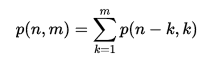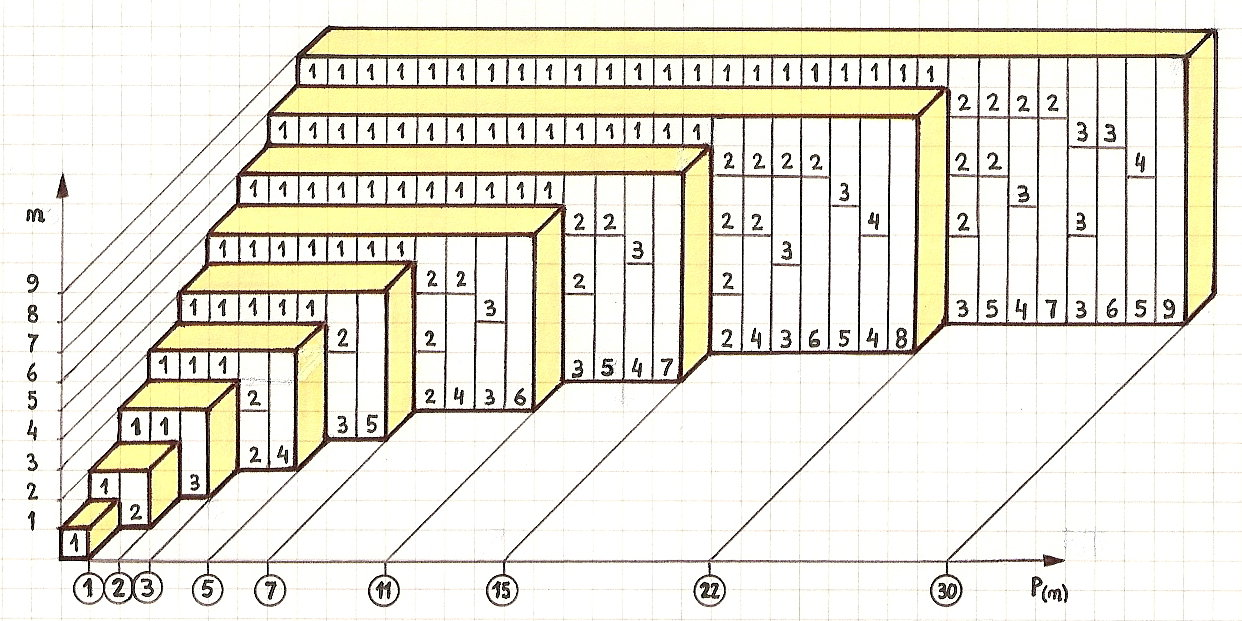# Basic Information of Integer Partition Algorithm

suggest change

The partition of an integer is a way of writing it as a sum of positive integers. For example, the partitions of the number 5 are:

• 5
• 4 + 1
• 3 + 2
• 2 + 2 + 1
• 2 + 1 + 1 + 1
• 1 + 1 + 1 + 1 + 1

Notice that changing the order of the summands will not create a different partition.

The partition function is inherently recursive in nature since the results of smaller numbers appear as components in the result of a larger number. Let p(n,m) be the number of partitions of n using only positive integers that are less than or equal to m. It may be seen that p(n) = p(n,n), and also p(n,m) = p(n,n) = p(n) for m > n.Example of Integer Partition Algorithm:Auxiliary Space: `O(n^2)`

Time Complexity: `O(n(logn))`